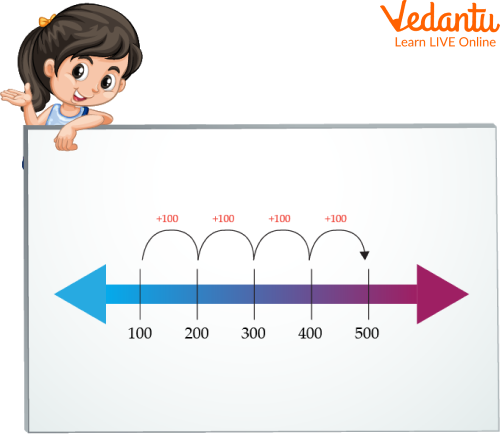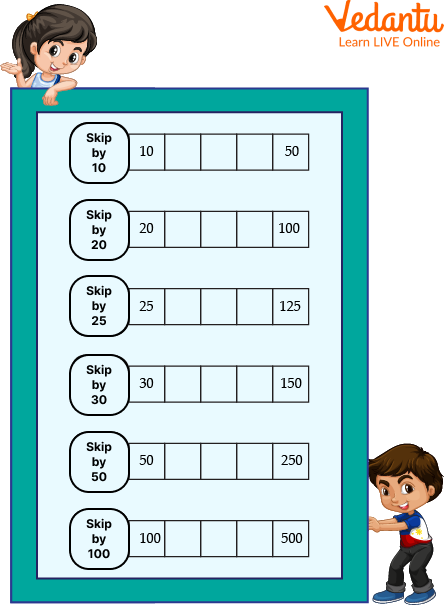Courses
Courses for Kids
Free study material
Free LIVE classes
More

# Skip Counting by 100## Introduction on Skip counting by 100

Skip counting by 100 is a method of learning to add numbers by 100. When we start learning the concept of addition, we start with skip counting and adding. When doing skip counting by 100, we keep adding the same number 100 to the previous number to get the next number in the series. The series obtained by skip counting by 100 starting with 0 looks like 0, 100, 200, 300, 400, and so on. This method of addition helps us in memorizing the counting numbers and the concept of addition.

The article talks about how skip counting is an essential skill for math and how important it is to learn it. In this article, we will learn to add numbers using the method of skip counting by 100.

## Steps to do Skip Counting by 100

To understand the process of skip counting by 100, we follow a certain set of steps to obtain the series of counting 100. Below is a list of steps we can follow to learn the process:

Step 1: Starting with the number 100, add 100 to the previous number to obtain the next number in the series. After 100, we obtain 100 + 100 = 200. This is the second number in the multiplication table of 100.

Step 2: To obtain the next number in the series, add 100 to 200, that is, 200 + 100 = 300. So, 300 is the next number in the series.

Step 3: As we add 100 to each previous number, we obtain the series as 100, 200, 300, 400, 500, 600, 700, and so on.

Step 4: 100, 200, 300, 400, 500, 600, 700, ... is the series of multiples of 100 that we obtained by skip counting by 100.

The following is the illustration of it:Ways of Doing Skip Counting by 100

## Skip Counting by 100 Chart

When skipping 100 natural numbers; we add 4 after each skip.

 Skip Counting by 100 Result 0+100 100 100+100 200 200+100 300 300+100 400 400+100 500 500+100 600 600+100 700 700+100 800 800+100 900 900+100 1000

## Skip Counting by 100 on Number Line

In this section; we will learn the concept of skip counting by 100 on a number line. We will make jumps to reach from one number to another.

Let us have a look at the number line below. Starting from the number 200, when skip counting by 100, the next number will be 200 + 100 = 300. So, we have landed on the number 300. Next, when we take another jump, we land on 300 + 100 = 400. Continuing in the same manner, we skip a number and land on the next number. So, the series continues like 200, 300, 400, 500, 600, 700, and so on.Skip Counting by 100 on Number Line

## Solved Examples

Here are the examples related to skip counting, which are as follows;

Q 1. Find the skip counting of 100 from 162.

Ans: To start the skip counting of 100 from 162, we need to add 100 to the next number.

So the first number will be 162.

Second number: 162 + 100 = 262

Third number: 262 + 100 = 362

And so on…

The pattern will become 162, 262, 362, 462, 562, …… and so on.

Q 3. Find the skip counting of 100 starting from 360.

Ans: To start the skip counting of 100 from 360, we need to add 100 to the next number.

So the first number will be 360.

Second number: 360 + 100 = 460

Third number: 460 + 100 = 560

And so on…

The pattern will become:

 360 460 560 660 760 860 960 1060 1160

## Skip Counting by 100 Worksheets

Here is the skip counting by 10 to 100 worksheets, which are as follows:Worksheet

## Summary

Skip counting is a great way to learn math, and it has been used in schools for decades. However, there are many different ways to skip count. This article will discuss the most common ones and show you how they work. Skip counting is a mathematical tool that can be used to find the sum of numbers. It is an efficient way to teach children how to count and can also be used as a mental exercise for adults. It is also a good way for them to practice their counting skills and ensure they get the correct answer.

This article concludes that Skip counting is an important tool for educators to use when teaching math skills and place value. In conclusion, skip counting is an easy and effective way to learn how to count. Children need to skip counting to prepare them for more difficult math concepts.

Last updated date: 21st Sep 2023
Total views: 75.9k
Views today: 2.75k

## FAQs on Skip Counting by 100

1. How can we use skip counting in math problems?

Numerous math issues can be solved using the skip-counting method. It is widely used to find the answer to subtraction, multiplication, and division problems. In this tutorial, we will learn how skip counting can be used to solve a variety of math problems.

2. How does skip counting help us learn math concepts better?

Skip counting is a way to practice and learn math concepts. It helps us become more familiar with numbers, which are the building blocks of math. It is a fun and easy way to practice our math skills. We can use it to learn addition, subtraction, multiplication and division. There are many ways we can use skip counting in our day-to-day life. We can play games like hopscotch and jump rope using skip counting or sing songs that involve skipping over words or phrases of the lyrics on every other count.

3. What exactly is skip counting?

Skip counting is more than just a speedy method for counting objects in equal groups or a method for learning multiplication facts. Skillfully and swiftly counting backwards is another essential component of developing number sense since it enables pupils to recognize patterns in numbers.

4. What are the benefits of using skip counting?

Skip counting is a mathematical technique that helps to teach children how to count by skipping numbers. This technique is a great way to teach children how to count, and this technique can be used for all ages. The benefits of using skip counting are that it can help children learn how to count, skip counting is fun and easy, and it is clearer than other techniques.